# Finding the coefficient of termsThis problem seem to bug many students, especially J1s who are doing Maclaurin’s series now. Many of the students are not sure how to find the coefficient of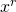. They wonder how to “see” it. So here, I’ll attempt to show a direct method that doesn’t require us to stare and think.

Considerand we are interested to find the coefficient of.

First of all, to applyformula, we rewrite to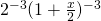. This step is based on indices and students should always check that the copied the correct power.

Now consider the formula above that is found in the MF15. We will take the coefficient ofthat’s found there, taking note thathere.

We will end of with the followingTake note we must copy thealongside too and we substitute thein and preserve the.

Simplifying, we have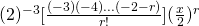Here we attempt to factoriseout, we need to figure how many (-1)’s we have here to factorise. To find out, we can taketo find out how many terms there are. Next, we try to simplify the factorials.Therefore, the coefficient is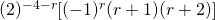.

Note:cannot be further simplified as we do not know ifis even or odd. In the event thatis even, then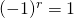and ifis odd, then. An example is if we considerinstead of, we observe that we will find a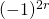which is equals to 1 since.

Not readable? Change text.